# Enneper Surface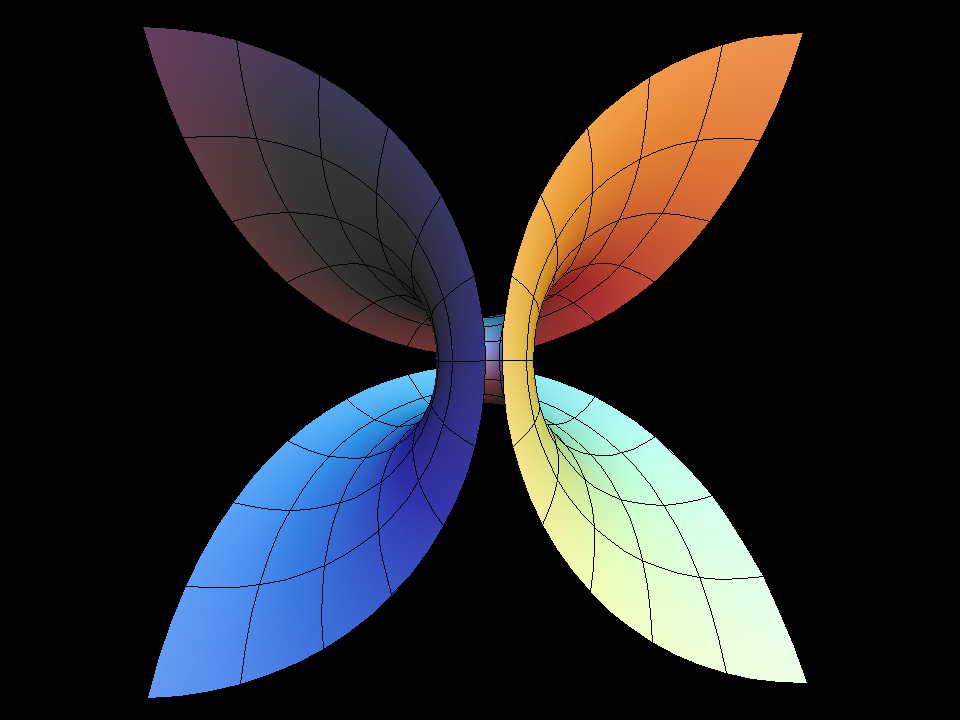Original Enneper surface, plotted with increasingly larger domain. The grids are Cartesian coordinate grid, and they happens also to be the principal curvature lines.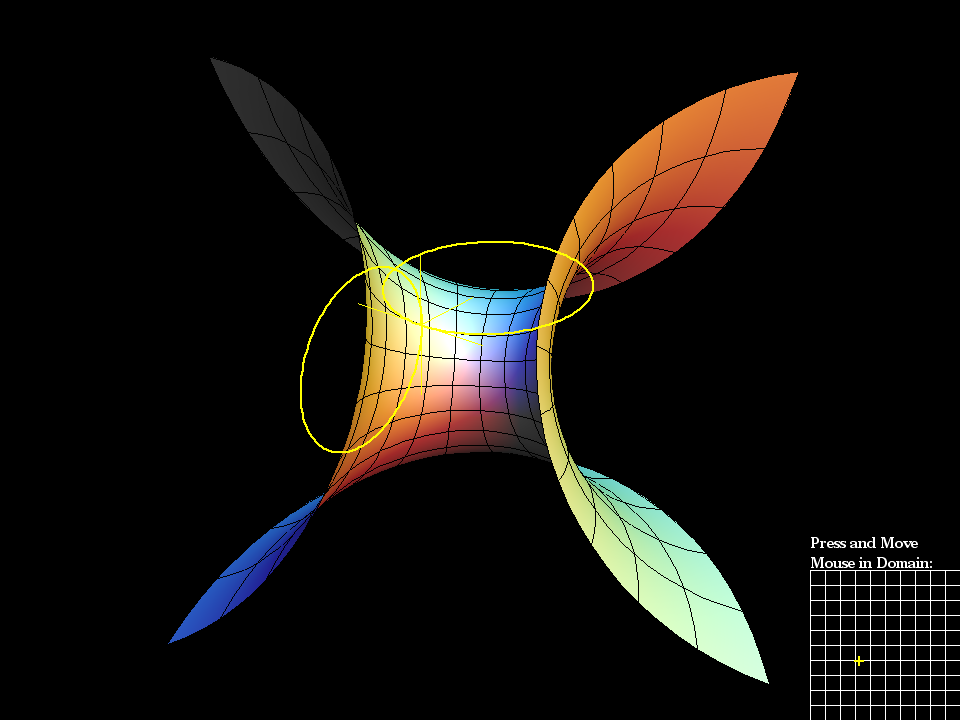Minimal surfaces are curved towards both sides in the same way: Observe that the curvature circles at a point are on different sides of the surface and have the same radius.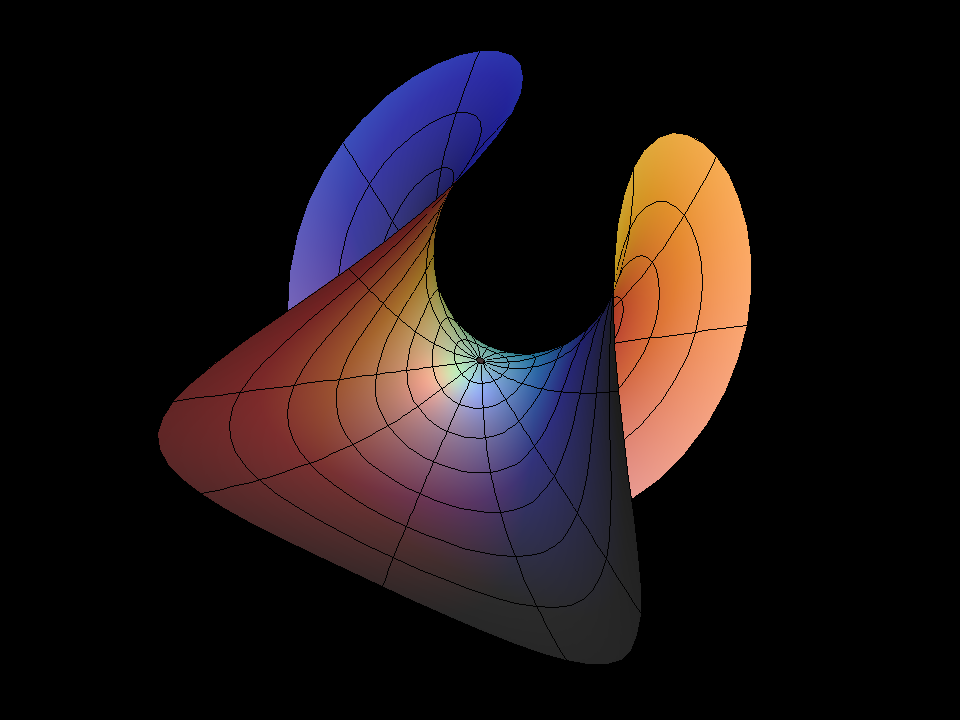In polar coordinates the symmetries of the surface are easier to see: Every second radial parameter line is a symmetry line (for reflection or 180 degrees rotation).

## Far Away View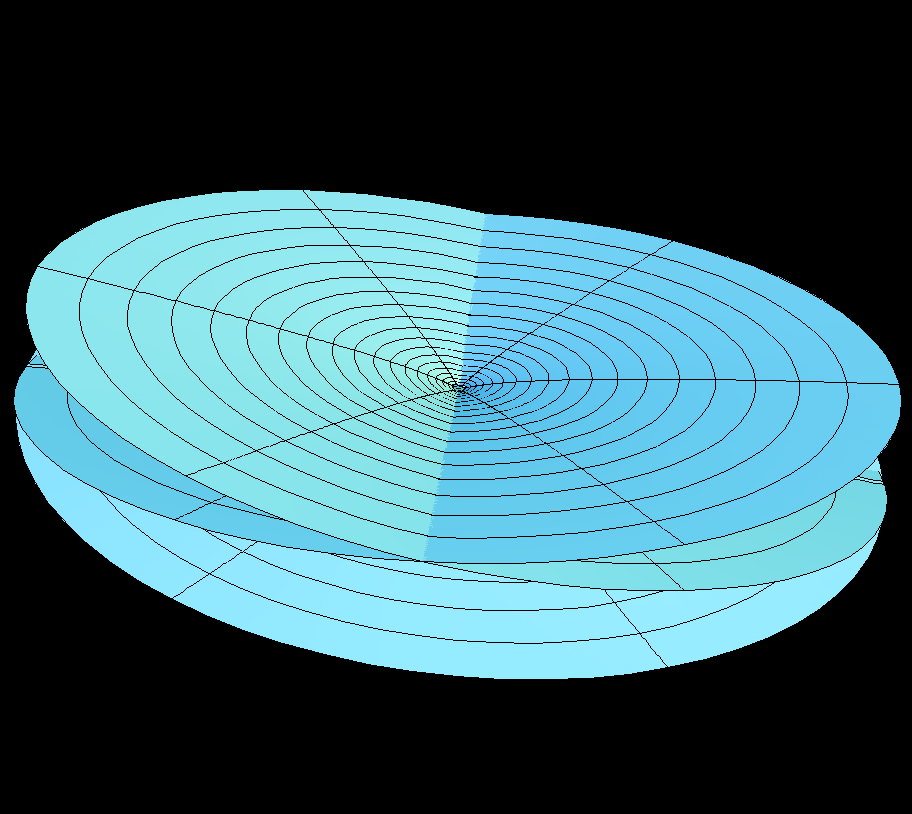Enneper Surface when viewed from far away

Here's how to visualize Enneper Surface looks like from far away.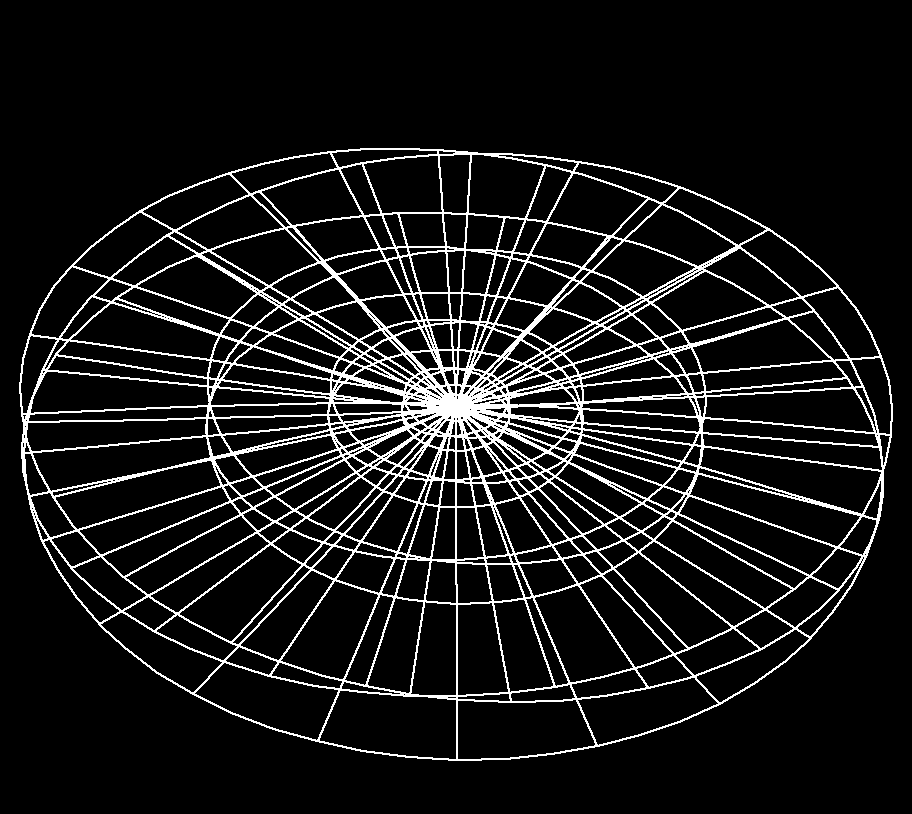Imagine, you run run around earth's equator 3 times: x(t) = R * cos(3t), y(t) = R * sin(3t), z(t) = 0, t goes from 0 to 2 π, R is radius of earth. Then “lift” it so that you have two maxima and 2 minima of z(t): x(t) = R * cos(3t), y(t) = R * sin(3t), z(t) = h * cos(2t) (h much smaller than R). Connect each point of this path to center of the earth. This surface is what Enneper looks like from far away.Enneper far away view (anaglyph)

## Enneper Surface with Higher Order Symmetry

Generalizations to Ennerper with higher symmetry were found 100 years later.

Enneper Surface Parametric Equations

```  x = u - u * u * u / 3 + u * v * v
y = v - v * v * v / 3 + v * u * u
z = u * u - v * v```

Enneper Surface is one of the finite total curvature immersions discovered in the 19th century. The generalizations to higher symmetry came in the 1980th.

The surfaces Wavy Enneper, Catenoid Enneper, Planar Enneper, and Double Enneper are finite total curvature minimal immersions of the once or twice punctured sphere — shown with standard polar coordinates. These surfaces illustrate how the different types of ends can be combined in a simple way.

The pure Enneper surfaces (Gauss(z)=z^k, k=ee+1) and the Planar Enneper have been re-discovered many times, because the members of the associate family are CONGRUENT surfaces (as can be seen in an associate family morphing) and the Weierstrass integrals integrate to polynomial (respectively) rational immersions.

Double Enneper was one of the early examples in which I joined two classical surfaces by a handle.

Formulas are taken from:

For a discussion of techniques for creating minimal surfaces with various qualitative features by appropriate choices of Weierstrass data, see:

• [KWH] H. Karcher, F. Wei, and D. Hoffman, The genus one helicoid, and the minimal surfaces that led to its discovery, in “Global Analysis in Modern Mathematics, A Symposium in Honor of Richard Palais' Sixtieth Birthday”, K. Uhlenbeck Editor, Publish or Perish Press, 1993
• [DHKW] U. Dierkes, S. Hildebrand, A. Kuster, and O. Wohlrab, Minimal Surfaces I, Grundlehren der math. Wiss. v. 295 Springer-Verlag, 1991

#### Related Surface of Enneper

Get red/blue stereo glasses from amazon

Enneper_Surface.pdf